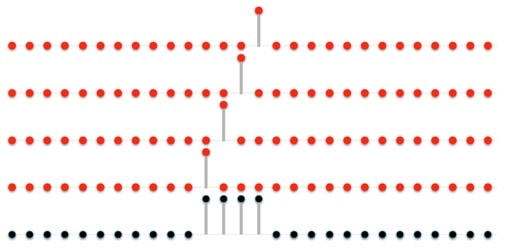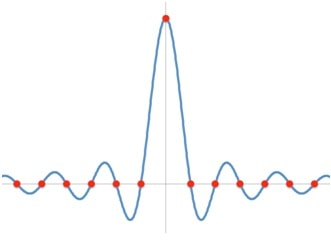# Cardinal Sinc

#### Issue 23

PCM encodes audio as a set of samples, each representing as closely as possible the actual amplitude of the audio waveform at some specific instant in time.  Recreating the audio is then a matter of using those samples to reconstruct the original waveform, data point by data point, because that’s what the data samples represent, isn’t it?  Actually, strictly speaking, it’s more complicated than that.

The reason for this was touched upon in my column a few weeks back, titled “50 Years After”.  It is a subtle point, but I think it’s worth understanding, so I’ll try to explain it in more detail.  In doing so, please bear in mind that for everything I am going to describe, I assume strict adherence to the Nyquist criterion, so that all waveforms contain no frequencies above one-half of the sampling rate.  When this strict criterion is breached, all bets are off.

When you want to recreate a waveform from a file full of samples, you need to ask what the samples actually represent, in isolation.  The totality of all of the samples is easily understood in the context of the ensemble, but what is the meaning, mathematically, of any one individual sample?  Only by fully understanding that meaning can you fully understand the process of using that sample – and its cohorts – to recreate the original waveform.

Let’s say we have a digitized audio waveform that comprises N samples.  We can consider that waveform to be the result of adding together N separate waveforms, each comprising sample values that are all zero, except for one non-zero value.  The non-zero values of each separate waveform each occupy different sample positions, and their values are those of the samples in the equivalent positions in the master waveform.  Summed together, all these ‘single-point’ waveforms add up to the original master waveform, as suggested by the diagram below:We have a term that we use to describe a ‘single-point’ waveform.  It is called an impulse, and it has a number of interesting properties (most of which I won’t be getting into in this column).

At this point I want to make a brief deviation, for reasons that will only become apparent later, in order to look at the process of extracting one individual sample from a data file.  I want to do this by placing a “window” over the entire data file, with a single gap in the window that allows you to view the data file only at the chosen sampling point.  This “window” function comprises a set of values that line up precisely with the contents of the data file.  Every value in the “window” function determines whether or not I can see through the window and view the sample behind it.  To extract just one sample, the window must be “open” at the one position that corresponds to the desired sample, and “closed” everywhere else.  In other words, my “window” function has a value of unity where the window is “open”, and zero where the window is “closed”.  It is, in fact, a unity-valued impulse function.

Mathematically, the process of applying a window function is called convolution.  By convolving an impulse function with a data file, the result is the specific sample at the location in the data file corresponding to the impulse.  Clearly, we can individually isolate each sample of the entire data file by convolving the data file with an appropriately aligned unity-valued impulse function.  Bear that in mind, and I’ll come back to it again later.

So, what kind of analog waveform does this digital impulse actually represent?   Consider the Sin() function (i.e. a sine wave).  It oscillates between positive and negative, crossing the x-axis (where it has a value of zero) at regularly-spaced intervals.  We’re looking for a function which behaves like the Sin() function, but somehow replaces one – and only one – of those zeros with a +1.  It turns out that our solution is a Sinc() function, related to the Sin() function as follows:

Sinc(x) = Sin(x)/x

When x=0, Sinc(0) evaluates to Sin(0)/0, which – surprisingly perhaps – works out be +1.  Hallelujah for that!  Here is what the central portion of the Sinc() function looks like, with the red dots indicating its value at certain regular sampling intervals:The oscillations of the Sinc() function go on infinitely in each direction, dying out slowly along the way.  From this diagram we can clearly see how our impulse data (the red dots) encode a Sinc() function (the blue curve), and how the samples get to be zero-valued everywhere except in the middle.  Therefore, by treating every individual sample in a digital audio file as a separate impulse waveform (as illustrated in my first diagram) every single one of them can be seen to actually encode its own Sinc() function.  You can also see from the spacing of the red dots that the particular Sinc() function we require is determined by the sample rate, and, aside from its amplitude, will therefore be the same every time.  In fact, the particular Sinc() function we want is Sinc(2πFst), where t is time, and Fs is the sample rate.

I can now take a final step – which is very important.  It is also perhaps tricky to grasp, so I apologize for that in advance.  Earlier, I mentioned convolution, which is using one function as a “window” through which to view another function.  We convolved the sampled digital waveform with the digital impulse function, and in doing so isolated an individual sample value.  It can easily be shown that the same is therefore true of their analog counterparts.  In other words, by convolving the equivalent analog waveform with a Sinc() function, we produce the exact same individual, isolated, sample value.  You might want to read that again …

What this actually shows is that the process of convolving a waveform with the function Sinc(2πFst) is the exact mathematical description for sampling that waveform at a sample rate of Fs.  This is a result of profound importance because it provides the crucial bridge – a lossless transformation – between the analog and digital worlds.

Knowing all this enables us to look at our sampled values, and figure out what we must do to transform them – losslessly – back into their original analog form.  The proper mathematical transformation involves taking every single sample value, and recreating its matching Sinc() function.  The sum of all those Sinc() functions will be the exact original waveform.  Unfortunately, though, recreating and summing Sinc() functions is a practical impossibility.  There is just no way to do that in any sort of real-world DAC.  Similarly, there is no way to convolve an analog waveform with a Sinc() function in a real-world ADC.  All of which brings us back down to earth again with a bump.  But it provides us with a framework against which we can measure all the things we can do, and determine how well we are doing them.

## Also From This Issue

Subscribe to Copper Magazine and never miss an issue.Stop by for a tour:
Mon-Fri, 8:30am-5pm MST

4865 Sterling Dr.
Boulder, CO 80301
1-800-PSAUDIO

# Join the hi-fi family

Stop by for a tour:
4865 Sterling Dr.
Boulder, CO 80301